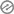# Three Dimensional Shapes

Class:2

Subject:MathematicsTopic-Three dimensional shapesContent:Identify object in our environment that are three dimensional shapes

Examples of cubesExamples of cuboids

Identify the flat faces,edges and vertices(corners)of cubes and cuboidsFeatures of cubes and cuboidsThree dimensional shapes are cubes,cuboids,cylinders and spheres

Examples of cubes are cubes of sugar,cubes of maggi and ludo dice

Examples of cuboids are mathematical set,toothpaste box,match box,e.t.cThe edges of a cube or cuboids is where the flat faces meet.The vertices is where the edge of cubes and cuboids meets.

Features of cubes and cuboids

CubeIt has 6 flat faces

It has 12 edges

It has a vertices(corners)

It has a square faceCuboids

It has 6 flat faces

It has 12 edges

It has 8 vertices

It has a rectangular face

Example

Take a cube of sugar and matchbox

Count the number of flat faces both objects have?How many flat faces do the objects have?

The cube of sugar and matchbox both have 6 flat faces.

Count the number of edges of cube of sugar and match box

How many edges do the objects have?The cube of sugar and matchbox both have 12 edges

Count the number of vertices the cube of sugar and matchbox

How many vertices do the objects have?

The cube of sugar and matchbox both have 8 verticesEvaluation Questions

Copy and complete each of the following statement1.A cube and cuboid each have

a) ___________________faces

b) ___________________edgec) ___________________vertices

2.Each face of cube is __________________3.Each face of cuboid is ___________________

4.List two objects in your environment that are cubes

___________and____________5.Name two objects in your environment that are cuboids

___________and____________

Sharing is Caring – Pass It On